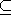# Chapter 5dD Convex Hulls and Delaunay Triangulations

Susan Hert and Michael Seel

## 5.1   Introduction

A subset Sd is convex if for any two points p and q in the set the line segment with endpoints p and q is contained in S. The convex hull of a set S is the smallest convex set containing S. The convex hull of a set of points P is a convex polytope with vertices in P. A point in P is an extreme point (with respect to P) if it is a vertex of the convex hull of P. A set of points is said to be strongly convex if it consist of only extreme points.

This chapter describes the class provided in CGAL for producing convex hull in arbitrary dimensions. There is an intimate relationship between the Delaunay triangulation of a point set S and the convex hull of lift(S): The nearest site Delaunay triangulation is the projection of the lower hull and the furthest site Delaunay triangulation is the upper hull. Here we also describe the companion class to the convex hull class that computes nearest and furthest site Delaunay triangulations.

## 5.2   dD Convex Hull

The class CGAL::Convex_hull_d<R> is used to represent the convex hull of a set of points in d-dimensional space. This class supports incremental construction of hulls, and provides a rich interface for exploration. There are also output routines for hulls of dimension 2 and 3.

The convex hull class is parameterized by a traits class that provides d-dimensional data types and predicates. The class Convex_hull_d_traits_3 adapts any low-dimensional standard kernel model e.g., Homogeneous<RT> or Cartesian<FT> for use with Convex_hull_d, where the dimension is fixed to three. The validity of the computed convex hull can be checked using the member funciton is_valid, which implements the algorithm of Mehlhorn et al.[MNS+96] to determine if the vertices of a given polytope constitute a strongly convex point set or not.

The implementation follows the papers [CMS93] and [BMS94].

## 5.3   Delaunay Triangulation

There is a class type with a thourough interface providing the construction and exploration of closest and furthest site Delaunay simplicial complexes in arbitrary higher dimension. The class CGAL::Delaunay_d<R, Lifted_R> provides an implementation via the lifting map to higher dimensional convex hulls. . The class supports incremental construction of Delaunay triangulations and various kind of query operations (in particular, nearest and furthest neighbor queries and range queries with spheres and simplices).